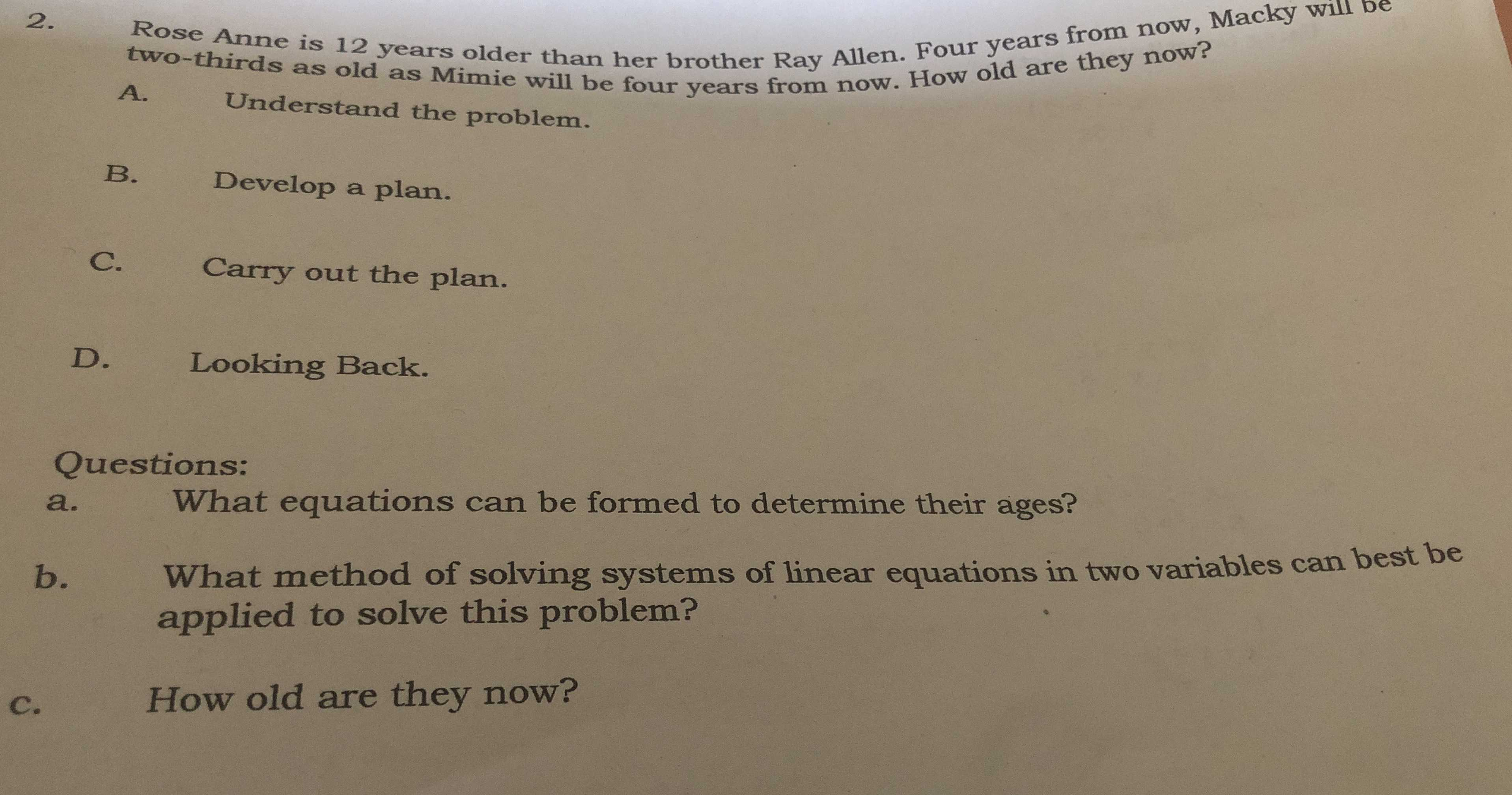### ¿Todavía tienes preguntas de matemáticas?

Pregunte a nuestros tutores expertos
Algebra
Pregunta2. Rose Anne is $$12$$ years older than her brother Ray Allen. Four years from now, Macky will be two-thirds as old as Mimie will be four years from now. How old are they now?

A. Anderstand the problem. B. Develop a plan. C. Carry out the plan. D. Looking Back

questions: What equations can be formed to determine their ages? b. What method of solving systems of linear equations in two variables can best be applied to solve this problem? c. How old are they now?

a+4=$$\frac{2}{3} b$$